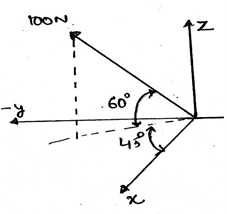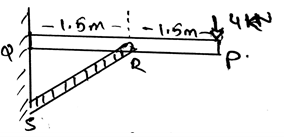Courses

# Test: Different Free Body Diagrams

## 15 Questions MCQ Test Engineering Mechanics | Test: Different Free Body Diagrams

Description
This mock test of Test: Different Free Body Diagrams for Mechanical Engineering helps you for every Mechanical Engineering entrance exam. This contains 15 Multiple Choice Questions for Mechanical Engineering Test: Different Free Body Diagrams (mcq) to study with solutions a complete question bank. The solved questions answers in this Test: Different Free Body Diagrams quiz give you a good mix of easy questions and tough questions. Mechanical Engineering students definitely take this Test: Different Free Body Diagrams exercise for a better result in the exam. You can find other Test: Different Free Body Diagrams extra questions, long questions & short questions for Mechanical Engineering on EduRev as well by searching above.
QUESTION: 1

### Just like the collinear forces for free body diagrams there are collinear couple and the net moment is taken out from it.

Solution:

Both of them are vector quantities. And both of them can be easily simplified. If taken in the vector form then the task is even easier. Thus it is not necessary for the force or the couple to be vector only, even if the magnitude is taken, the simplification is done in the 2D.

QUESTION: 2

### The simplification of the forces on the free body diagrams is done as __________

Solution:

A particular system of the rules is followed that is if the upward direction is taken as positive then the downward direction is taken as negative. This is same as done with the couple in the 2D. That is the forces can be easily simplified. If taken in the vector form then the task is even easier.

QUESTION: 3

### In the figure given below, calculate the value of cosγ. (γ is the angle made by the resultant vector and the z-axis)Solution:

Well, the question is just a tricky one. Just subtract 60 from 90, you will get the γ, which is 30. And then apply the cosine formula for getting the components of respective axis.

QUESTION: 4

The system of the collinear and the parallel force in free body diagrams are simplified as _________

Solution:

The simplification is easy. The simplified force system gives us a net force and the parallel force system gives us a simplified force, and then we add it vectorially. Thus the vector addition at the last can be easily done and thus both can be simplified.

QUESTION: 5

The couple is a scalar quantity and the force is vector quantity and hence only force can be simplified in free body diagrams.

Solution:

Both of them are vector quantities. And both of them can be easily simplified. If taken in the vector form then the task is even easier. Thus it is not necessary for the force or the couple to be vector only, even if the magnitude is taken, the simplification is done in the 2D.

QUESTION: 6

The couple is simplified easily by the help of right hand rule in free body diagrams. But the forces simplification is not possible as there is no such system of rules so that the forces can be simplified.

Solution:

Both of them are vector quantities. And both of them can be easily simplified. If taken in the vector form then the task is even easier. Thus it is not necessary for the force or the couple to be vector only, even if the magnitude is taken, the simplification is done in the 2D. Forces are simplified on the basis of the algebra.

QUESTION: 7

The couple and the other two force systems in free body diagrams can be easily simplified.

Solution:

Both of them are vector quantities. And both of them can be easily simplified. If taken in the vector form then the task is even easier. Thus it is not necessary for the force or the couple to be vector only, even if the magnitude is taken, the simplification is done in the 2D.

QUESTION: 8

Determine the vertical components of the reaction on the beam at pin Q.Solution:

The summation of the forces needs to be zero. So does the summation of the moments need to zero. But talking about the angles, they not needed to zero. But the forces which are acting at particular angles, must needed to be equal to zero. The basic need of the forces to make the body in equilibrium.

QUESTION: 9

The force and the couple can’t be simplified together as one is the cause and the other is the effect in free body diagrams.

Solution:

Both of them are vector quantities. And both of them can be easily simplified. If taken in the vector form then the task is even easier. Thus it is not necessary for the force or the couple to be vector only, even if the magnitude is taken, the simplification is done in the 2D.

QUESTION: 10

What is a collinear system of forces for free body diagrams?

Solution:

The force system having all the forces emerging from a point is called the collinear system of force. This is a type of system of the force, which is easy in the simplification. This is because as the forces are the vector quantity, the vector math is applied and the simplification is done.

QUESTION: 11

The main moto of the free body diagrams is to:

Solution:

The main moto of the free body diagrams is to reduce the calculation. The forces are simplified and the couples too are simplified. This gives us the total force and the total moment acting on the body. This gives us a single value of the respective thing and hence reduces the labour.

QUESTION: 12

The couple moment and the force is divided to get the distance of the axis from the point of action of the force in free body diagrams.

Solution:

The couple is the cross product of the force and the perpendicular distance between the forces. And if the division of the both is done than the resulting quantity is the distance only. But care must be taken that the product is the cross product and hence sine angle must be divided too to get the distance.

QUESTION: 13

The simplification of the couple is done for free body diagrams on the basis of the:

Solution:

The couple is simplified by taking the direction of the rotation of the body as positive or negative. That is if the clockwise direction is positive then the anti-clockwise direction is taken as negative. If the couple is a vector it can be easily simplified by taking the components.

QUESTION: 14

Principle of transmissibility for free body diagrams is:

Solution:

Principle of transmissibility states that the force acting on the body is a sliding vector. That is it can be applied at any point of the body. It will give the same effect as if applied at any point other that the specific point. Thus the answer.

QUESTION: 15

Just like the collinear force system for free body diagrams there is a system of the parallel forces.

Solution:

There is a system so called the parallel system of the forces. This is another type of the forces. The simplification of this type of the forces is also very easy. This means that the forces in a certain direction is taken as positive and in the other direction is negative and hence the simplification is done.